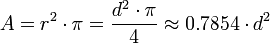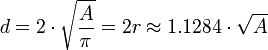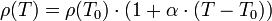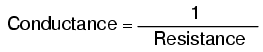Deutsche VersionConversion and calculation − cross section < > diameter

Cable diameter to circle cross-sectional area and vice versa ●

Round electric cable, conductor, wire, cord, string, wiring, and rope

 Cross section is just a two-dimensional view of a slice through an object. An often asked question: How can you convert the diameter of a round wire d = 2 × r to the circle cross section surface or the cross-section area A (slice plane) to the cable diameter d? Why is the diameter value greater than the area value? Because that's not the same. Resistance varies inversely with the cross-sectional area of a wire.   The required cross-section of an electrical line depends on the following factors: 1) Rated voltage. Net form. (Three-phase (DS) / AC (WS)) 2) Fuse - Upstream backup = Maximum permissible current (Amp) 3) On schedule to be transmitted power (kVA) 4) Cable length in meters (m) 5) Permissible voltage drop (% of the rated voltage) 6) Line material. Copper (Cu) or aluminum (Al)

 Wire diameter d unit | Cross-sectional area A unit2 | ↓ | ↓ | Cross-sectional area A unit2 | Wire diameter d unit |

The "unit" is usually millimeters but it can also be inches, feet, yards, meters (metres),
or centimeters, when you take for the area the square of that measure.

Litz wire (stranded wire) consisting of many thin wires need a 14 % larger diameter compared to a solid wire.

 Cross sectional area is not diameter.

 Cross section is an area. Diameter is a linear measure. That cannot be the same.   The cable diameter in millimeters is not the cable cross-section in square millimeters.The cross section or the cross sectional area is the area of such a cut. It need not necessarily have to be a circle.   Commercially available wire (cable) size as cross sectional area: 0.75 mm2, 1.5 mm2, 2.5 mm2, 4 mm2, 6 mm2, 10 mm2, 16 mm2.

Calculation of the cross section A, entering the diameter d = 2 r:r = radius of the wire or cable
d = 2
r = diameter of the wire or cable

Calculation of the diameter d = 2 r, entering the cross section A:The conductor (electric cable)

 There are four factors that affect the resistance of a conductor: 1) the cross sectional area of a conductor A, calculated from the diameter d 2) the length of the conductor 3) the temperature in the conductor 4) the material constituting the conductor

 There is no exact formula for the minimum wire size from the maximum amperage. It depends on many circumstances, such as for example, if the calculation is for DC, AC or even for three-phase current, whether the cable is released freely, or is placed under the ground. Also, it depends on the ambient temperature, the allowable current density, and the allowable voltage drop, and whether solid or litz wire is present. And there is always the nice but unsatisfactory advice to use for security reasons a thicker and hence more expensive cable. Common questions are about the voltage drop on wires.

Voltage drop Δ V

The voltage drop formula with the specific resistance (resistivity) ρ (rho) is:

 Δ V = I × R = I × (2 × l × ρ / A)

I = Current in ampere
l = Wire (cable) length in meters (times 2, because there is always a return wire)
ρ = rho, electrical resistivity (also known as specific electrical resistance or volume
resistivity) of copper = 0.01724 ohm×mm2/m (also Ω×m)
(Ohms for
l = 1 m length and A = 1 mm2 cross section area of the wire)     ρ = 1 / σ
A = Cross section area in mm2
σ = sigma, electrical conductivity (electrical conductance) of copper = 58 S·m/mm2

 Quantity of resistanceR = resistance Ω ρ = specific resistance Ω×m l = double length of the cable m A = cross section mm2

The derived SI unit of electrical resistivity ρ is Ω × m, shortened from the clear Ω × mm² / m.
The reciprocal of electrical resistivity is electrical conductivity.

Electrical conductivity and electrical resistivity κ or σ = 1/ρ
Electrical conductance and electrical resistance
ρ = 1/κ = 1/σ

 Electrical  conductor Electrical conductivity  Electrical conductance Electrical resistivity  Specific resistance silver σ = 62 S·m/mm² ρ = 0.0161 Ohm∙mm²/m copper σ = 58 S·m/mm² ρ = 0.0172 Ohm∙mm²/m gold σ = 41 S·m/mm² ρ = 0.0244 Ohm∙mm²/m aluminium σ = 36 S·m/mm² ρ = 0.0277 Ohm∙mm²/m constantan σ = 2.0 S·m/mm² ρ = 0.5000 Ohm∙mm²/m

Difference between electrical resistivity and electrical conductivity

 The conductance in siemens is the reciprocal of the resistance in ohms.

 To use the calculator, simply enter a value. The calculator works in both directions of the ↔ sign.

 Electrical conductivity σ  S × m / mm² ↔ Specific elec. resistance ρ  Ohm × mm² / m σ = 1 / ρ ρ = 1 / σ siemens S = 1/Ω or ohm Ω = 1/S
 The value of the electrical conductivity (conductance) and the specific electrical resistance (resistivity) is a temperature dependent material constant. Mostly it is given at 20 or 25°C.

Resistance = resistivity x length / area

 The specific resistivity of conductors changes with temperature. In a limited temperature range it is approximately linear:where α is the temperature coefficient, T is the temperature and T0 is any temperature, such as T0 = 293.15 K = 20°C at which the electrical resistivity ρ (T0) is known.

Convert resistance to electrical conductance
Conversion of reciprocal siemens to ohms
1 ohm [Ω] = 1 / siemens [1/S]
1 siemens [S] = 1 / ohm [1/Ω]

 To use the calculator, simply enter a value. The calculator works in both directions of the ↔ sign.

 Resistance R  ohms Ω ↔ Electrical conductance G  siemens S R = 1 / G G = 1 / R

1 millisiemens = 0.001 mho = 1000 ohms

 Mathematically, conductance is the reciprocal, or inverse, of resistance:The symbol for conductance is the capital letter "G" and the unit is the mho, which is "ohm" spelled backwards. Later, the unit mho was replaced by the unit Siemens − abbreviated with the letter "S".

Calculator: Ohm's law

Table of typical loudspeaker cables

 Cable diameter d 0.798 mm 0.977 mm 1.128 mm 1.382 mm 1.784 mm 2.257 mm 2.764 mm 3.568 mm Cable nominal cross section A 0.5 mm2 0.75 mm2 1.0 mm2 1.5 mm2 2.5 mm2 4.0 mm2 6.0 mm2 10.0 mm2 Maximum electrical current 3 A 7.6 A 10.4 A 13.5 A 18.3 A 25 A 32 A -

Always consider, the cross section must be made larger with higher power and higher length of
the cable, but also with lesser impedance. Here is a table to tell the possible power loss
.

 Cable length in m Section    in mm2 Resistance in ohm Power loss at Damping factor at Impedance 8 ohm Impedance 4 ohm Impedance 8 ohm Impedance 4 ohm 1 0.75 0.042 0.53% 1.05% 98 49 1.50 0.021 0.31% 0.63% 123 62 2.50 0.013 0.16% 0.33% 151 75 4.00 0.008 0.10% 0.20% 167 83 2 0.75 0.084 1.06% 2.10% 65 33 1.50 0.042 0.62% 1.26% 85 43 2.50 0.026 0.32% 0.66% 113 56 4.00 0.016 0.20% 0.40% 133 66 5 0.75 0.210 2.63% 5.25% 32 16 1.50 0.125 1.56% 3.13% 48 24 2.50 0.065 0.81% 1.63% 76 38 4.00 0.040 0.50% 1.00% 100 50 10 0.75 0.420 5.25% 10.50% 17 9 1.50 0.250 3.13% 6.25% 28 14 2.50 0.130 1.63% 3.25% 47 24 4.00 0.080 1.00% 2.00% 67 33 20 0.75 0.840 10.50% 21.00% 9 5 1.50 0.500 6.25% 12.50% 15 7 2.50 0.260 3.25% 6.50% 27 13 4.00 0.160 2.00% 4.00% 40 20

The damping factor values show, what remains of an accepted damping factor of 200
depending on the cable length, the cross section, and the impedance of the loudspeaker.

Conversion and calculation of cable diameter to AWG
and AWG to cable diameter in mm - American Wire Gauge

 Wire diameter d mm | AWG number | ↓ | ↓ | AWG number | Wire diameter d mm |
 The gauges we most commonly use are even numbers, such as 18, 16, 14, etc. If you get an answer that is odd, such as 17, 19, etc., use the next lower even number.   AWG stands for American Wire Gauge and refers to the strength of wires. These AWG numbers show the diameter and accordingly the cross section as a code. They are only used in the USA. Sometimes you find AWG numbers also in catalogues and technical data in Europe.

American Wire Gauge - AWG Chart

AWG
number
46 45 44 43 42 41 40 39 38 37 36 35 34
Diameter
in inch
0.0016 0.0018 0.0020 0.0022 0.0024 0.0027 0.0031 0.0035 0.0040 0.0045 0.0050 0.0056 0.0063
Diameter (Ø)
in mm
0.04 0.05 0.05 0.06 0.06 0.07 0.08 0.09 0.10 0.11 0.13 0.14 0.16
Cross section
in mm2
0.0013 0.0016 0.0020 0.0025 0.0029 0.0037 0.0049 0.0062 0.0081 0.010   0.013   0.016   0.020

AWG
number
33 32 31 30 29 28 27 26 25 24 23 22 21
Diameter
in inch
0.0071 0.0079 0.0089 0.0100 0.0113 0.0126 0.0142 0.0159 0.0179 0.0201 0.0226 0.0253 0.0285
Diameter (Ø)
in mm
0.18 0.20 0.23 0.25 0.29 0.32 0.36 0.40 0.45 0.51 0.57 0.64 0.72
Cross section
in mm2
0.026 0.032 0.040 0.051 0.065 0.080 0.10 0.13 0.16 0.20 0.26 0.32 0.41

AWG
number
20 19 18 17 16 15 14 13 12 11 10 9 8
Diameter
in inch
0.0319 0.0359 0.0403 0.0453 0.0508 0.0571 0.0641 0.0719 0.0808 0.0907 0.1019 0.1144 0.1285
Diameter (Ø)
in mm
0.81 0.91 1.02 1.15 1.29 1.45 1.63 1.83 2.05 2.30 2.59 2.91 3.26
Cross section
in mm2
0.52 0.65 0.82 1.0 1.3 1.7 2.1 2.6 3.3 4.2 5.3 6.6 8.4

AWG
number
7 6 5 4 3 2 1 0
(1/0)
(0)
00
(2/0)
(-1)
000
(3/0)
(-2)
0000
(4/0)
(-3)
00000
(5/0)
(-4)
000000
(6/0)
(-5)
Diameter
in inch
0.1443 0.1620 0.1819 0.2043 0.2294 0.2576 0.2893 0.3249 0.3648 0.4096 0.4600 0.5165 0.5800
Diameter (Ø)
in mm
3.67 4.11 4.62 5.19 5.83 6.54 7.35 8.25 9.27 10.40 11.68 13.13 14.73
Cross section
in mm2
10.6 13.3 16.8 21.1 26.7 33.6 42.4 53.5 67.4 85.0 107.2 135.2 170.5

How are high frequencies damped by the length of the cable?